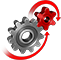# WolframSystemModeler

## Buck-Boost Converter

Buck-boost converters are used both to step up voltage from a lower level to a higher level and to step down voltage from a higher level to a lower level. Buck-boost converters can be found in applications where the supply voltage changes over time, such as battery-powered applications.

To run this example, you'll need

### The Model

A buck-boost converter is a switched-mode power converter that uses two switches (usually a diode and a transistor), an inductor, and a capacitor to convert direct current voltage from a lower to a higher level, or the other way around. When the switch is turned on, the current goes through the inductor and increases the energy there. When the switch is turned off, the inductor current goes through the load and the diode. A filter capacitor is added to smooth out the output voltage.A model of a buck-boost converter created in SystemModeler from standard components.

### Operation and Duty RatioThe switch is turned on, the inductor is charged, and the capacitor supplies the load.The switch is turned off, and the inductor supplies the load together with the capacitor.
The relationship for the voltage conversion ratiois given by, where the duty ratiois the ratio of time the switch is on in one cycle. With zero it is off all the time, and with one it is on for the whole cycle. With abelow 0.5, the converter will step down the voltage, and above 0.5 the converter will step up the voltage.Voltage is stepped up from 24 to 36 voltage with a duty ratioof 0.6.Voltage is stepped down from 24 to 16 voltage with a duty ratioof 0.4.
The buck-boost converter allows for both higher and lower voltage levels. This increased flexibility compared to the buck and boost converters comes at a price: higher circuit complexity and higher stress on the components.

### Detect Discontinuous Mode

If the output load is below a certain threshold, the inductor current will drop to zero during an interval in the switching cycle; the converter then enters discontinuous conducting mode (DCM). If the duty ratio is not changed during DCM, the output voltage can climb to dangerously high levels and the components will see increased stress and wear. Here the Wolfram Language is used to detect DCM.In DCM the current goes to zero within the switching cycle.

Analyze Simulation Results in the Wolfram Language

Calculate different metrics, such as time in DCM and voltage ripple in the downloadable example.

Model and Simulate Efficient Switched‐Mode Power Converters

The hybrid differential equation-solving capabilities of SystemModeler make it easy to create high‐fidelity models of switched DC-to-DC converters.

## Wolfram System Modeler

Questions? Comments? Contact a Wolfram expert »